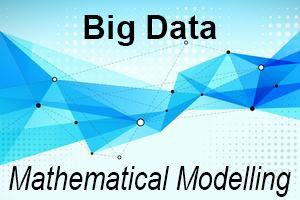# Free Online Course: Big Data - Mathematical Modelling

When:

Course starts 17 September 2018

Where:

Online## course3-mooc.jpg

Learn how mathematics underpins big data analysis and develop your skills.

Mathematics is everywhere, and with the rise of big data it becomes a useful tool when extracting information and analysing large datasets. We begin by explaining how maths underpins many of the tools that are used to manage and analyse big data.

We show how very different applied problems can have common mathematical aims, and therefore can be addressed using similar mathematical tools. We then introduce three such tools, based on a linear algebra framework: eigenvalues and eigenvectors for ranking; graph Laplacian for clustering; and singular value decomposition for data compression.

This course is part of the Big Data Analytics program with FutureLearn, which will enable you to gain the big data analytics skills that are in demand today.